## Number System

### Number System

A number system is a way to represent numbers or quantities and symbols. We are using thebase-10 number system, which is also called decimal.

Decimal is a base or radix 10 number system. This means that the symbols or numerals torepresent any quantity. These symbols are called digits. The ten symbols are 1, 2, 3, 4, 5, 6, 7, 8, 9, 0.

Other common number systems are of base-2 (binary), use of 0, 1

base-8 (octal), use of 0, 1, 2, 3, 4, 5, 6, 7

base-16 (hexadecimal).0, 1, 2, 3, 4, 5, 6, 7, 8, 9, A, B, C,D, E, F

where A stands for 10, B stands for 11, C stands for 12, D stands for 13, E stands for 14 and F stands for 15.

Type of Numbers.

Natural Numbers :-Counting numbers i.e. 1, 2, 3, 4, 5,…………….

Whole numbers:- Natural numbers and 0 i.e 0, 1, 2, 3, 4, 5,…………

Integers:- All the whole numbers including negative numbers. i. e. 0, ±1,±2, ±3, ±4, ±5, … … … … …

Rational Numbers :- All numbers of the form, p and q are integers, but q Ex:- 4, -8,, 0, 32,…….

Irrational Numbers:- Irrational numbers are the opposite of rational numbers, nonterminating and non-recurring numbers.

Ex-,,, 2,+0 ……………..

Real Numbers:- Real numbers comprise the full spectrum of numbers. They can be in any form, natural numbers, whole numbers, integers, rational numbers, rational or irrational numbers. They can be represented by a number line.

– ———————————– —-0——————————————

Ex- 7, -2, 0, 4, 8,, ……..etc.

Even Numbe:-A number which is a multiple of 2., Ex-2, 4. 6. 8, 10, ……….

Odd Number:- An odd number is one that can not divide completely by 2.

Ex- 1, 3, 5, 7, 9,…….

Prime Number:- A prime number is a number, which can be divided by 1 and itself. 2 is the only even number which is also a prime number.

Example of prime numbers- 2, 3, 5, 7, 11, 13, 17,……..

Composite Number:- A composite number is a number which can be divided by number of factors other than 1 and itself.

Ex- 4, 6, 8, 9, 10, 14,………..

Co-Primes or relatively prime:- A pair of numbers not having any common factors other than 1 or -1. Or we can say the greatest common factor is 1 or -1.

Ex-15 and 28 are co-prime, because the factor of 15 are 1,3,5,15 and the factor of 28 is 1, 2,4,7, 14, 28. They have no common factors except 1.

Twin prime:- A pair of prime numbers that differ by 2 i.e two consequtive odd prime nos.Ex(3,5),(5, 7), (11, 13),…….

Imaginary Numbers:- A number of the form ,,n is natural number.

Imaginary ‘i’=,

Place value and face value- In decimal system the value of a digit depends on it’s place or position in the number. Each place has a value of 10 times the place to the right. Face value of a number is the value of the number without regard to where it is in relation to another number. Ex- 7 always has a face value of 7, whether it belongs to 247 or 742. How ever the place value includes the position of the number in another number. Ex. In number 4732, 7 hasa place value of 700 but have a face vlue of just 7.

Names of digits according to their place value.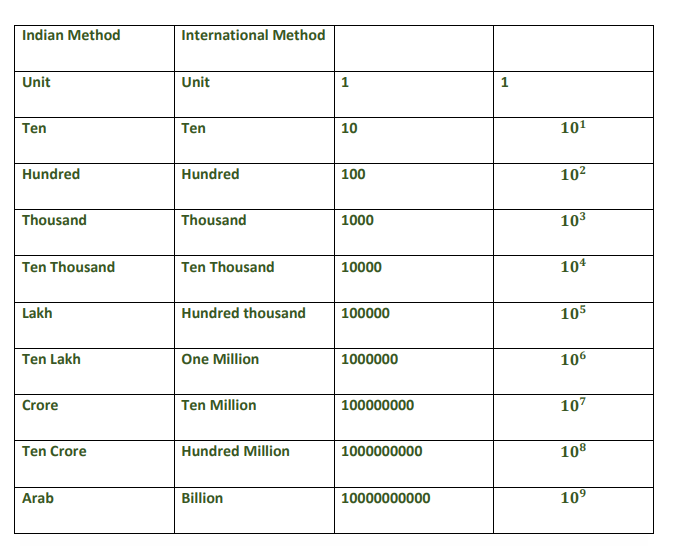Partnerships

When two or more people joins hands with a common goal to attain profits. Every partner invests either time, money or his patents to help partnership firm to reap profits

Raj invested Rs 76000 in a business. After few months Monty joined him and invests Rs 57000. At the end of year both of them share the profits at the ratio of 2:1. After how many months Monty joined Raj?

Solution – We can simply compute per month investment of both partnership

Raj invested Rs 76,000 for 12 months and Monty invested Rs 57,000 for x months.

Now 76000 × 12 / 57000 × x = 2 :1

⇒ 76 × 12 / 2 = 57x

⇒ x = 8

So Monty invested his money for 8 months and he joined after 4 months.

Question 2

A and B started a business by investing money in ratio of 5:6. C joined them after few months by sharing an amount equal to B’s share. At the end of year 20% profit was earned which was equal equal to Rs 98,000. How much money was invested by C ?

Solution –

= First of all we will calculate the weighted ratios

⇒ A = 5 × 12 = 60

⇒ B = 6 × 12 = 72

⇒ C = 6 × 6 = 36

Total investment at the end of year = 98000 × 100/20 = Rs 4,90,000

⇒ Investment by C = 490000 × 36 / 168 × 2 = Rs 210000

Question 3

A, B and C shared profits in ratio of 5:7:8. They partnered for 14 months, 8 months and 7 months respectively. What was he ratio of their investments ?

Solution – Simply multiply profit sharing ratio with investment ratio to ge investment amount ratio.

Let X is the total investment

⇒14 x = 5

⇒ 8 x = 7

⇒ 7x = 8 ⇒

Final investment ratio = 20 : 49 : 64

Question 3

Sita and Geeta started a business by investing Rs. 120000 and Rs.135000 respectively. Find the share of each out of an annual profit of Rs. 35700.

Solution :–     Sita          Gita

⇑                ⇑

Ratio of their share =120000:135000=8:9

Sum of the parts of the ration =8+9=17

Sita’s share =35700 ×  8/17=Rs.16800

Gata’share=35700 × 9/17=Rs.18900

When investors are there for the different duration of time in the business , then the investments are calculated for a unit of time by having ( investment x number of units of time) . Now gain or loss is divided in the ratio of these investments.

Question 4.

Ravi started a business by investing Rs.36000. After 3 months Shayam joined him by investing Rs.36000.Find the share of each in the annual profit of Rs. 37100.

Solution –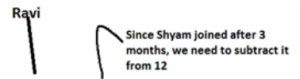Ratio of their share =36000 × 12 :36000 × 9=4:3

Sum of the parts of the ration =8+9=17

Sita’s share =37100 ×  8/7=Rs.21200

Gata’share=37100 × 3/17=Rs.15900

Now let us do some questions:

Question 5.

Sony , Mony and Tony start a business each investing Rs.20000. After 5 months Sony withdrew Rs.5000,Mony withdrew Rs.4000 and Tony added Rs.6000 more. At the end of theyear, a total profit Of Rs.69900 was recorded. Find the share of each?

Solution – Ratio of the investments of sony, Mony and Tony =20000 × 5 + 15000 × 7 :20000 ×5+26000 × 7

Explanation:    since sony invest for 5 months is Rs.20000 and then Withdraws Rs 5000 after 5 months so investment is now 20000-5000= 15000 for remaining months that is 12-5=7.Slmilar is the case with Mony. In case of Tony why we do not subtract is because he is not withdrawing Rather he is investing 6000 extra after 5 months.

=205000:212000:292000=205:212:282

Sum parts of the ratio=205+212+282=699

Sony’s share=69000 × 205/699 = Rs.20500

Mony’s share=69900 × 212/699 = Rs.21200

Tony’s share=69900 × 282/699 = Rs.28200

Question 6.

Sheela started a business with Rs. 21000 and is joined afterwords by Reema with Rs 36000. After how many months did Reema join if the profits at the end of the year are divided equally?

Let Reema joins after x months.

Then Reema’s invstments was for (12-x) months

Since the profits was divided equally

21000 x 12 =3600  (12-x)

12-x=21000 x 12/36000

12-x=7

-x=7-12

-x=-5

X=5

So Reema joined after 5 months

Question 7.

X and Y invested in a business. They earned some profit which they divided in the ratio of 2:3. If Xinvested Rs. 40000. Find the amount invested by Y?

Solution –

Let Y invests Rs.m

Ratio of their capitals = 40000 x 12 : m x 12 = 40000 : m

And we know that according to the investments, prfit ratio is decided

So 2:3 = 40000:m

2/3  = 40000/m

M=40000 x 3/2 =Rs 60000

Question 8.

A , B , C contract a work for Rs .1100.A and B together are to do 7/11 of the work . What is C’s share ?

Solution –

We know that A and B share = 7/11 C

‘s share = 1-7/11 = 4/11

C ‘s share = Rs.1100 x 4/11 = Rs.400

Question 9.

Mohinder and Surinder entered into a partnership investing Rs. 12000 and Rs. 9000 respectively . After 3months , Sudhir joined them with an investment of Rs.15000. What is the share of Sudhir in a half yearlyprofit of Rs. 9500?

Solution –

Mohinder:surinedr:sudhir =12000 x 6 : 15000 x 3 =8:6:5

Explanation : Now this time we need to calculated the profit half yearly that is why 6 is multiplication in case of Mohuinedr and surinfer. In case of sudhir, since he comes after 3 months so number of monts is 6-3=3

Sum of parts of Ratio = 8+6+5=19

Shaer of sudhir = 9500 x 5/10=Rs.9500

Question 10.

In a partnership , A invests 1/6 of the capital for 1/6 of the time , B invests 1/3 of the capital for 1/3 ofthe time and C , the rest of the capital for the whole time. What is the share of B in the profit f Rs. 4600.

Solution – Let the total capital is Rs.m and the whole period is y months.

A invests m/6 for y/6 months

B invests m/3 for y/3 months

C invests =m-(m/6+m/3)=m/2 for y months

A : B : C =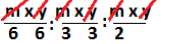= 1/36 :1/9/:1/2

=36 x 1/36 : x 1/9 : 36 x 1/2

LCM of 36,9 and 2 is 36, so we multiply each Ratio by 36 to make it a proper ratio.

=1 : 2 : 18

Sum of the parts = 1+4+18 = 23

B ‘s share = 4600 x/ 23 = Rs.800

Question 11.

Three partners shared the profit in a business in the ratio 5 : 7 : 8. They had partnered for 14 months, 8months and 7 months respectively. What was the ratio of their investments?

Solution – Let the first partner invests Rs x for 14 months, second partner invests Rs.y for 8 months, Rs zfor 7 months.

14x : 8y : 7z =5 : 7 : 8=98x = 40y

Y===112 x =35 z

z ==X : y: z = x ::=20x : 49x :64x

=20 : 49 : 64

Question 12.

Ajay and Sahil enter into a partnership investing Rs. 50000 and Rs. 40000, respectively. They agree to share profits in the ratio of their capitals. Find the share of Ajay in a profit of Rs. 22500 after one

(a) Rs. 12500

(b) Rs. 9500

(c) Rs 10500

(d) None of these

Question 13.

Navdeep, Rahul and Raj entered into a partnership Navdeep invested Rs. 16000 for 9 months. Rahul invested Rs. 12000 for 6 months and Raj invested Rs. 8000 for 12 months. At the end of a year there was a profit of Rs. 26000. Find the share of Rahul
in the profit.

(a) Rs. 8000

(b) Rs. 7500

(c) After 4.5 months

(d) None of these

Question 16.

A, B and C invested capitals in the ratio 3:5:9; the timing of their investments being in the ratio 2:3 1. In what ratio would their profits be distributed?

(a) 2:5:3

(b) 3:2:5

(c) 7:5:3

(d) None of these

Question 17.

A, B and C start a business. If the ratio of their periods of investments are 2:3:6 and their profits are in the ratio of 4:5:6, then the ratio of capitals of A, B and C is

(a) 6:8:10

(b) 12 10:6

(c) 10: 12:6

(d) None of these

Question 18.

A, B, C and D enter into partnership, A subscribes 1/3 of the capital, B 1/4, C 1/5 and D the rest. What is the share of D out of a profit of Rs. 6000?

(a) Rs. 2000

(b) Rs. 1600

(c) Rs. 1200

(d) Rs. 1300

Question 19.

A and B started a business with initial investments in the ratio 5:7. If after one year their profits were in the ratio 1:2 and the period for A’s investment was 7 months, B invested the money for

(a) 6 months

(b) 9 months

(c) 10 months

(d) 4 months

Question 20.

A, B, C enter into a partnership with shares in the 7/2:4/3:6/5 ratio After 4 months, A increase his share by 50%. If the total profit at the end of one year be Rs. 21600, then B’s share in the profit is

(a) Rs. 2100

(b) Rs. 2400

(c) Rs. 3600

(d) Rs, 4000

Question 21.

B is a sleeping partner and A working. A puts in Rs.5000 and B puts in 6000, A received 12.5% of profit for managing the business and rest is divided in proportion to their capitals. A’s share of profit in a profit of Rs. 880 is

(a) Rs. 350

(b) Rs. 400

(c) Rs. 420

(d) Rs. 460

Question 22.

A starts business with a capital of Rs. 1200. B and C join with some investments after 3 and 6 months, respectively. If at the end of a year, the profit is divided in the ratio 2:3:5 respectively, what is B’s investment in the business?

(a) Rs. 2400

(c) Rs. 3600

(b) Rs. 1800

(d) Rs. 6000

Solutions

Ans. 12. (a)

Here, C1= 50000, C2 = 40000 and P =22500

Here time period of investment is constant

So ratio in which profit will be divided is

C1: C2 ⇒ 50k : 40k = 5 : 4

Now Ajay’s Share = 5 /9 ✕ 22500

= 5/9 Rs. 12500

Ans. 13. (c)

Here, C1= 16000, C2 = 12000 and P =8000

t 1 = 9, t2 =6, t3= 12 and p= 26000

ratio in which profit will be divided is

p1 : P2 : P3 will be C1t1 : C2t2 : C3t3

16000 9 : 12000  6 : 8000   12

P1 : p2 : p3 = 6:3:4

Rahul’s Share = 6000.

Ans. 14. (c)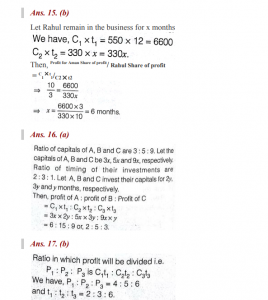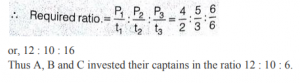. Ans. 18. (d)

D ‘s Capital =1-=Profit ratio of A,B,C,D is∴20 : 15 : 12 : 13

∴Share of D =×   Rs.600=Rs1300

Ans. 19. (c)

Let investments  of A and B respectively be 5x and

7x and period of B’s investment be y months.

Then ,=⇒ y=10

Ans. 20. (d)

Given ratio =::=105 : 40 : 36

Let the initially invest  Rs . 105,Rs. 40 and Rs. 36,

Respectively

Ratio of investments

=  [105  ×  4+(150% of 105) 8] : (40× 12 ): (36 × 12)

=1680:480 : 432= 35 : 10 :9

B ‘s share =Rs=Rs4000.

Ans. 21.(d)

A ‘s share for managing the business

=12.5 % of Rs. 880 =Rs.110

Remaining profit =Rs .770

Profit ratio of A and B =5: 6

A ‘s share =of Rs.770 =Rs . 350.

A ‘s total profit = Rs 350 +Rs.110 =Rs.460

Ans. 22. (a)

Profit ratio of A,B and C is

(1200 × 12) : (x × 9) : (y × 6)=2:3:5

Taking first and second terms we get

1200 ×  12: 9x= 2:3

1200 ×12  ×3=9x  ×2

X== 2400

Important questions and solutions

Question 1

Sheela started a business in 2009 by investing Rs.50,000. She invested Rs. 20,000 as additional amount in 2010 and her friend Devi joined her with an amount of Rs.70,000. Sheela invested another Rs. 20,000 in 2011 and Anu joined them with Rs. 70,000. At the end of these 3 years, they earned a profit of Rs. 3,00,000. Find Devi’s share?

a) Rs.1,00,000 b) Rs.1,25,000 c) Rs.75,000 d) Rs.1,50,000

Answer : a) Rs.1,00,000

Solution :

Sheela invested Rs.50,000 for 12 months, Rs.(50000 + 20000) for 12 months and Rs.(50000 + 20000 + 20000) for 12 months.

i.e., she invested Rs.50,000 for 12 months, Rs.70000 for 12 months and Rs.90000 for 12 months.

Devi invested Rs. 70000 for 2 years; i.e., Rs.70000 for 24 months

And, Anu invested Rs.70000 for 1 year; i.e., Rs. 70000 for 12 months.

Their investing ratio:

Sheela : Devi : Anu = (50,000 x 12 + 70000 x 12 + 90000 x 12):(70000 x 24):(70000 x 12)

= (25,20,000):(16,80,000):(8,40,000) = 252:168:84 = 3:2:1

Total profit for 3 years = Rs.3,00,000

Therefore, Devi’s share = Rs.(3,00,000 x 2 /(3+2+1)) = Rs.(3,00,000 x 2/6) = Rs.1,00,000

Question 2

Rahul and Rafick start a business with investments of Rs. 2500 and Rs. 2250 respectively. After 2 months, Rahul takes out Rs.1250 from his capital. After 1 more month, Rafick takes out Rs.750 of his capital while Ramesh joins them with a capital of Rs. 3500. At the end of 6 months, they earn a profit of Rs. 2540. Which of the following is the share of each member in the profit?

a) Rahul – Rs. 700, Rafick – Rs. 950, Ramesh – Rs. 890

b) Rahul – Rs. 900, Rafick – Rs. 750, Ramesh – Rs. 890

c) Rahul – Rs. 800, Rafick – Rs. 900, Ramesh – Rs. 840

d) Rahul – Rs. 840, Rafick – Rs. 800, Ramesh – Rs. 900

Answer : c) Rahul – Rs. 800, Rafick – Rs. 900, Ramesh – Rs. 840

Solution :

Initial investment of Rahul = Rs.2500.

After 2 months he withdraw Rs.1250 from his capital.

Therefore, we have, Rahul invested Rs.2500 for 2 months and Rs.(2500-1250=) 1250 for 4 months.

Rafick invested Rs. 2250 for 3 months and Rs.(2250-750=) 1500 for 3 months.

And, Ramesh invested Rs.3500 for 3 months;

Their investing ratio:

Rahul:Rafick:Ramesh

= (2500×2 + 1250×4):(2250×3 + 1500×3):(3500×3) = (10,000):(11,250):(10,500) = 1000:1125:1050 = 40:45:42

Total profit for 6 months = Rs.2540

Therefore, Rahul’s share = Rs.(2540 x 40/(40+45+42)) = Rs.(2540 x 40/127) = Rs.800

Rafick’s share = Rs.(2540 x 45/127) = Rs.900

Ramesh’s share = Rs.(2540 x 42/127) = Rs.840

Hence, the answer is option c.

Question 3

Akash and Bharati started a business by investing some amount in the ratio of 4 : 7. Karthick joined them after six months with an amount equal to that of Bharati. What will be their profit ratio for a period of 1 year?

a) 8 : 14 : 7 b) 4 : 7 : 5 c) 4 : 7 : 7 d) 8 : 7 : 5

Answer : a) 8:14:7

Solution :

Given that their ratio of investment is 4:7

Let the initial investment of Akash and Bharati be 4X and 7X.

Therefore, Akash : Bharati : Karthick = (4X × 12):(7X × 12):(7X × 6)

= 48 : 84 : 42

= 16:28:14 = 8:14:7.

Question 4

Mr.X starts a business with Rs.7000 and after 10 months, Mr.Y joins with Mr.X by investing certain amount. At the end of 2 years, if 2:3 is the proportion of the profit then Mr.Y’s contribution in the capital is:

a) Rs.15000 b) Rs.16000 c) Rs.17000 d) Rs.18000

Answer : d) Rs.18000

Solution :

Let Mr.Y’s capital be Rs.P

Mr.X’s investment = Rs.7000 for 24 months

Mr.Y’s investment = Rs.P for 14 months

we know that, Profit ratio = investing ratio

i.e., (7000 x 24):(P x 14) = 2:3

168000 : 14P = 2:3

12000 : P = 2:3

12000/P = 2/3

P = 3 x 12000 / 2 = 18000

Required answer is Rs.18,000

Percentage

Percentage means converting a given factor to a denominator of 100.

Example- 89 % means= 0.89

EXample- Convert the fraction 3/5 in to percentage fraction. i.e . 3/5 = (3/5×100)% = 60%.

Permutations and Combinations

1. Factorial Notation:

Let n be a positive integer. Then, factorial n, denoted n! is defined as:

n! = n(n – 1)(n – 2) … 3.2.1.

Examples:

1. We define 0! = 1.

2. 4! = (4 x 3 x 2 x 1) = 24.

3. 5! = (5 x 4 x 3 x 2 x 1) = 120.

2. Permutations:

The different arrangements of a given number of things by taking some or all at a time, are called permutations.

‘Examples:

1. All permutations (or arrangements) made with the letters a, b, c by taking two at a time are (ab, ba, ac, ca, bc, cb).

2. All permutations made with the letters a, b, c taking all at a time are: ( abc, acb, bac, bca, cab, cba)

3. Number of Permutations:

Number of all permutations of n things, taken r at a time, is given by:

nPr = n(n – 1)(n – 2) … (n – r + 1) =Examples:

1. 6P2 = (6 x 5) = 30.

2. 7P3 = (7 x 6 x 5) = 210.

Cor. number of all permutations of n things, taken all at a time = n!.

4. An Important Result:

If there are n subjects of which p1 are alike of one kind; p2 are alike of another kind; p3 are alike of third kind and so on and pr are alike of r thkind, such that (p1 + p2 + … pr) = n.

Then, number of permutations of these n objects is =Combinations:

Each of the different groups or selections which can be formed by taking some or all of a number of objects is called a combination.

Examples:

1. Suppose we want to select two out of three boys A, B, C. Then, possible selections are AB, BC and CA. Note: AB and BA represent the same selectio

2. All the combinations formed by a, b, c taking ab, bc, ca.

3. he only combination that can be formed of three letters a, b, c taken all at a time is abc.

4. Various groups of 2 out of four persons A, B, C, D are: AB, AC, AD, BC, BD, CD. Note that ab ba are two different permutations but they represent the same combination.

Number of Combinations:

The number of all combinations of n things, taken r at a time is: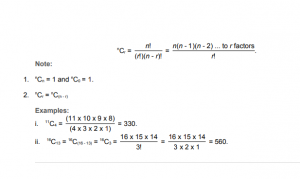1. From a group of 7 men and 6 women, five persons are to be selected to form a committee so that at least 3 men are there on the committee. In how many ways can it be done?

A. 564

B. 645

C. 735

D. 756

E. None of these

Explanation:

We may have (3 men and 2 women) or (4 men and 1 woman) or (5 men only).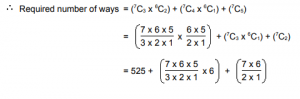= (525 + 210 + 21)

= 756.

2. In how many different ways can the letters of the word ‘LEADING’ be arranged in such a way that the vowels always come together?

A. 360

B. 480

C. 720

D. 5040

E. None of these

Explanation:

The word ‘LEADING’ has 7 different letters.

When the vowels EAI are always together, they can be supposed to form one letter.

Then, we have to arrange the letters LNDG (EAI).

Now, 5 (4 + 1 = 5) letters can be arranged in 5! = 120 ways.

The vowels (EAI) can be arranged among themselves in 3! = 6 ways.

∴Required number of ways = (120 x 6) = 720.

3. In how many different ways can the letters of the word ‘CORPORATION’ be arranged so that the vowels always come together?

A. 810

B. 1440

C. 2880

D. 50400

E. 5760

Explanation:

In the word ‘CORPORATION’, we treat the vowels OOAIO as one letter.

Thus, we have CRPRTN (OOAIO).

This has 7 (6 + 1) letters of which R occurs 2 times and the rest are different.

Number of ways arranging these letters = 7! = 2520. 2!

Now, 5 vowels in which O occurs 3 times and the rest are different, can be arranged in 5! = 20 ways. 3!

∴ Required number of ways = (2520 x 20) = 50400.

4. Out of 7 consonants and 4 vowels, how many words of 3 consonants and 2 vowels can be formed?

A. 210

B. 1050

C. 25200

D. 21400

E. None of these

Explanation:

Number of ways of selecting (3 consonants out of 7) and (2 vowels out of 4)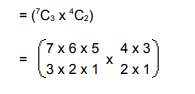= 210.

Number of groups, each having 3 consonants and 2 vowels = 210.

Each group contains 5 letters.= 5!

Number of ways of arranging

5 letters among themselves

= 5 x 4 x 3 x 2 x 1

= 120.

∴ Required number of ways = (210 x 120) = 25200.

5. In how many ways can the letters of the word ‘LEADER’ be arranged?

A. 72

B. 144

C. 360

D. 720

E. None of these

Explanation:

The word ‘LEADER’ contains 6 letters, namely 1L, 2E, 1A, 1D and 1R.

Required number of ways == 360.

6. In a group of 6 boys and 4 girls, four children are to be selected. In how many different ways can they be selected such that at least one boy should be there?

A. 159

B. 194

C. 205

D. 209

E. None of these

Explanation:

We may have (1 boy and 3 girls) or (2 boys and 2 girls) or (3 boys and 1 girl) or (4 boys).

∴ Required number of ways

= (6C1 x 4C3) + (6C2 x 4C2) + (6C3 x 4C1) + (6C4)

= (6C1 x 4C1) + (6C2 x 4C2) + (6C3 x 4C1) + (6C2)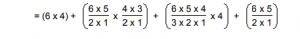= 209.

7. How many 3-digit numbers can be formed from the digits 2, 3, 5, 6, 7 and 9, which are divisible by 5 and none of the digits is repeated?

A. 5

B. 10

C. 15

D. 20

Explanation:

Since each desired number is divisible by 5, so we must have 5 at the unit place. So, there is 1 way of doing it.

The tens place can now be filled by any of the remaining 5 digits (2, 3, 6, 7, 9). So, there are 5 ways of filling the tens place.

The hundreds place can now be filled by any of the remaining 4 digits. So, there are 4 ways of filling it

∴Required number of numbers = (1 x 5 x 4) = 20.

8. In how many ways a committee, consisting of 5 men and 6 women can be formed from 8 men and 10 women?

A. 266

B. 5040

C. 11760

D. 86400

E. None of these

Explanation: Required number of ways = (8C5 x 10C6) = (8C3 x 10C4) = 8 x 7 x 6 x 10 x 9 x 8 x 7 3 x 2 x 1 4 x 3 x 2 x 1 = 11760. 9. A box contains 2 white balls, 3 black balls and 4 red balls. In how many ways can 3 balls be drawn from the box, if at least one black ball is to be included in the draw? A. 32 B. 48 C. 64 D. 96 E. None of these Answer: Option C Explanation: We may have(1 black and 2 non-black) or (2 black and 1 non-black) or (3 black). Required number of ways = (3C1 x 6C2) + (3C2 x 6C1) + (3C3) = 3 x 6 x 5 + 3 x 2 x 6 + 1 2 x 1 2 x 1 = (45 + 18 + 1) = 64. 10. In how many different ways can the letters of the word ‘DETAIL’ be arranged in such a way that the vowels occupy only the odd positions? A. 32 B. 48 C. 36 D. 60 E. 120 Answer: Option C Explanation: There are 6 letters in the given word, out of which there are 3 vowels and 3 consonants. Let us mark these positions as under: (1) (2) (3) (4) (5) (6) Now, 3 vowels can be placed at any of the three places out 4, marked 1, 3, 5. Number of ways of arranging the vowels = 3P3 = 3! = 6. Also, the 3 consonants can be arranged at the remaining 3 positions. Number of ways of these arrangements = 3P3 = 3! = 6. Total number of ways = (6 x 6) = 36. 11. In how many ways can a group of 5 men and 2 women be made out of a total of 7 men and 3 women? A. 63 B. 90 C. 126 D. 45 E. 135 Answer: Option A Explanation: Required number of ways = (7C5 x 3C2) = (7C2 x 3C1) = 7 x 6 x 3 = 63. 2 x 1 12. How many 4-letter words with or without meaning, can be formed out of the letters of the word, ‘LOGARITHMS’, if repetition of letters is not allowed? A. 40 B. 400 C. 5040 D. 2520 Answer: Option C Explanation: ‘LOGARITHMS’ contains 10 different letters. Required number of words = Number of arrangements of 10 letters, taking 4 at a time. = 10P4 = (10 x 9 x 8 x 7) = 5040. 13. In how many different ways can the letters of the word ‘MATHEMATICS’ be arranged so that the vowels always come together? A. 10080 B. 4989600 C. 120960 D. None of these Answer: Option C Explanation: In the word ‘MATHEMATICS’, we treat the vowels AEAI as one letter. Thus, we have MTHMTCS (AEAI). Now, we have to arrange 8 letters, out of which M occurs twice, T occurs twice and the rest are different. Number of ways of arranging these letters = 8! = 10080. (2!)(2!) Now, AEAI has 4 letters in which A occurs 2 times and the rest are different. Number of ways of arranging these letters = 4! = 12. 2! Required number of words = (10080 x 12) = 120960. 14. In how many different ways can the letters of the word ‘OPTICAL’ be arranged so that the vowels always come together? A. 120 B. 720 C. 4320 D. 2160 E. None of these Answer: Option B Explanation: The word ‘OPTICAL’ contains 7 different letters. When the vowels OIA are always together, they can be supposed to form one letter. Then, we have to arrange the letters PTCL (OIA). Now, 5 letters can be arranged in 5! = 120 ways. The vowels (OIA) can be arranged among themselves in 3! = 6 ways. Required number of ways = (120 x 6) = 720. Pipes and Cistern 1. Inlet: A pipe connected with a tank or a cistern or a reservoir, that fills it, is known as an inlet. Outlet: A pipe connected with a tank or cistern or reservoir, emptying it, is known as an outlet. 2. If a pipe can fill a tank in x hours, then: part filled in 1 hour = 1 . x 3. If a pipe can empty a tank in y hours, then: part emptied in 1 hour = 1 . y 4. If a pipe can fill a tank in x hours and another pipe can empty the full tank in y hours (where y > x), then on opening both the pipes, then the net part filled in 1 hour = 1 – 1 . x y 5. If a pipe can fill a tank in x hours and another pipe can empty the full tank in y hours (where x > y), then on opening both the pipes, then the net part emptied in 1 hour = 1 – 1 . y x 1. Three pipes A, B and C can fill a tank from empty to full in 30 minutes, 20 minutes, and 10 minutes respectively. When the tank is empty, all the three pipes are opened. A, B and C discharge chemical solutions P,Q and R respectively. What is the proportion of the solution R in the liquid in the tank after 3 minutes? A. 5 11 B. 6 11 C. 7 11 D. 8 11 Answer: Option B Explanation: Part filled by (A + B + C) in 3 minutes = 3 1 + 1 + 1 = 3 x 11 = 11 . 30 20 10 60 20 Part filled by C in 3 minutes = 3 . 10 Required ratio = 3 x 20 = 6 . 10 11 11 2. Pipes A and B can fill a tank in 5 and 6 hours respectively. Pipe C can empty it in 12 hours. If all the three pipes are opened together, then the tank will be filled in: A. 1 13 hours 17 B. 2 8 hours 11 C. 3 9 hours 17 D. 4 1 hours 2 Answer: Option C Explanation: Net part filled in 1 hour 1 + 1 – 1 = 17 . 5 6 12 60 The tank will be full in 60 hours i.e., 3 9 hours. 17 17 3. A pump can fill a tank with water in 2 hours. Because of a leak, it took 2 hours to fill the tank. The leak can drain all the water of the tank in: A. 4 1 hours 3 B. 7 hours C. 8 hours D. 14 hours Answer: Option D Explanation: Work done by the leak in 1 hour = 1 – 3 = 1 . 2 7 14 Leak will empty the tank in 14 hrs. 4. Two pipes A and B can fill a cistern in 37 minutes and 45 minutes respectively. Both pipes are opened. The cistern will be filled in just half an hour, if the B is turned off after: A. 5 min. B. 9 min. C. 10 min. D. 15 min. Answer: Option B Explanation: Let B be turned off after x minutes. Then, Part filled by (A + B) in x min. + Part filled by A in (30 -x) min. = 1. x 2 + 1 + (30 – x). 2 = 1 75 45 75 11x + (60 -2x) = 1 225 75 11x + 180 – 6x = 225. x = 9. 5. A tank is filled by three pipes with uniform flow. The first two pipes operating simultaneously fill the tank in the same time during which the tank is filled by the third pipe alone. The second pipe fills the tank 5 hours faster than the first pipe and 4 hours slower than the third pipe. The time required by the first pipe is: A. 6 hours B. 10 hours C. 15 hours D. 30 hours Answer: Option C Explanation: Suppose, first pipe alone takes x hours to fill the tank . Then, second and third pipes will take (x -5) and (x – 9) hours respectively to fill the tank. 1 + 1 = 1 x (x – 5) (x – 9) x – 5 + x = 1 x(x – 5) (x – 9) (2x – 5)(x – 9) = x(x – 5) x 2 – 18x + 45 = 0 (x – 15)(x – 3) = 0 x = 15. [neglecting x = 3] 6. Two pipes can fill a tank in 20 and 24 minutes respectively and a waste pipe can empty 3 gallons per minute. All the three pipes working together can fill the tank in 15 minutes. The capacity of the tank is: A. 60 gallons B. 100 gallons C. 120 gallons D. 180 gallons Answer: Option C Explanation: Work done by the waste pipe in 1 minute = 1 – 1 + 1 15 20 24 = 1 – 11 15 120 = – 1 . [-ve sign means emptying] 40 Volume of 1 part = 3 gallons. 40 Volume of whole = (3 x 40) gallons = 120 gallons. 7. A tank is filled in 5 hours by three pipes A, B and C. The pipe C is twice as fast as B and B is twice as fast as A. How much time will pipe A alone take to fill the tank? A. 20 hours B. 25 hours C. 35 hours D. Cannot be determined E. None of these Answer: Option C Explanation: Suppose pipe A alone takes x hours to fill the tank. Then, pipes B and C will take x and x hours respectively to fill the tank. 2 4 1 + 2 + 4 = 1 x x x 5 7 = 1 x 5 x = 35 hrs. 8. Two pipes A and B together can fill a cistern in 4 hours. Had they been opened separately, then B would have taken 6 hours more than A to fill the cistern. How much time will be taken by A to fill the cistern separately? A. 1 hour B. 2 hours C. 6 hours D. 8 hours Answer: Option C Explanation: Let the cistern be filled by pipe A alone in x hours. Then, pipe B will fill it in (x + 6) hours. 1 + 1 = 1 x (x + 6) 4 x + 6 + x = 1 x(x + 6) 4 x 2 – 2x – 24 = 0 (x -6)(x + 4) = 0 x = 6. [neglecting the negative value of x] 9. Two pipes A and B can fill a tank in 20 and 30 minutes respectively. If both the pipes are used together, then how long will it take to fill the tank? A. 12 min B. 15 min C. 25 min D. 50 min Answer: Option A Explanation: Part filled by A in 1 min = 1 . 20 Part filled by B in 1 min = 1 . 30 Part filled by (A + B) in 1 min = 1 + 1 = 1 . 20 30 12 Both pipes can fill the tank in 12 minutes. 10. Two pipes A and B can fill a tank in 15 minutes and 20 minutes respectively. Both the pipes are opened together but after 4 minutes, pipe A is turned off. What is the total time required to fill the tank? A. 10 min. 20 sec. B. 11 min. 45 sec. C. 12 min. 30 sec. D. 14 min. 40 sec. Answer: Option D Explanation: Part filled in 4 minutes = 4 1 + 1 = 7 . 15 20 15 Remaining part = 1 – 7 = 8 . 15 15 Part filled by B in 1 minute = 1 20 1 : 8 :: 1 : x 20 15 x = 8 x 1 x 20 = 10 2 min = 10 min. 40 sec. 15 3 The tank will be full in (4 min. + 10 min. + 40 sec.) = 14 min. 40 sec. 11. One pipe can fill a tank three times as fast as another pipe. If together the two pipes can fill the tank in 36 minutes, then the slower pipe alone will be able to fill the tank in: A. 81 min. B. 108 min. C. 144 min. D. 192 min. Answer: Option C Explanation: Let the slower pipe alone fill the tank in x minutes. Then, faster pipe will fill it in x minutes. 3 1 + 3 = 1 x x 36 4 = 1 x 36 x = 144 min. 12. A large tanker can be filled by two pipes A and B in 60 minutes and 40 minutes respectively. How many minutes will it take to fill the tanker from empty state if B is used for half the time and A and B fill it together for the other half? A. 15 min B. 20 min C. 27.5 min D. 30 min Answer: Option D Explanation: Part filled by (A + B) in 1 minute = 1 + 1 = 1 . 60 40 24 Suppose the tank is filled in x minutes. Then, x 1 + 1 = 1 2 24 40 x x 1 = 1 2 15 x = 30 min. 13. A tap can fill a tank in 6 hours. After half the tank is filled, three more similar taps are opened. What is the total time taken to fill the tank completely? A. 3 hrs 15 min B. 3 hrs 45 min C. 4 hrs D. 4 hrs 15 min Answer: Option B Explanation: Time taken by one tap to fill half of the tank = 3 hrs. Part filled by the four taps in 1 hour = 4 x 1 = 2 . 6 3 Remaining part = 1 – 1 = 1 . 2 2 2 : 1 :: 1 : x 3 2 x = 1 x 1 x 3 = 3 hours i.e., 45 mins. 2 2 4 So, total time taken = 3 hrs. 45 mins. 14. Three taps A, B and C can fill a tank in 12, 15 and 20 hours respectively. If A is open all the time and B and C are open for one hour each alternately, the tank will be full in: A. 6 hours B. 6 2 hours 3 C. 7 hours D. 7 1 hours 2 Answer: Option C Explanation: (A + B)’s 1 hour’s work = 1 + 1 = 9 = 3 . 12 15 60 20 (A + C)’s hour’s work = 1 + 1 = 8 = 2 . 12 20 60 15 Part filled in 2 hrs = 3 + 2 = 17 . 20 15 60 Part filled in 6 hrs = 3 x 17 = 17 . 60 20 Remaining part = 1 – 17 = 3 . 20 20 Now, it is the turn of A and B and 3 part is filled by A and B in 1 hour. 20 Total time taken to fill the tank = (6 + 1) hrs = 7 hrs. 15. Three pipes A, B and C can fill a tank in 6 hours. After working at it together for 2 hours, C is closed and A and B can fill the remaining part in 7 hours. The number of hours taken by C alone to fill the tank is: A. 10 B. 12 C. 14 D. 16 Answer: Option C Explanation: Part filled in 2 hours = 2 = 1 6 3 Remaining part = 1 – 1 = 2 . 3 3 (A + B)’s 7 hour’s work = 2 3 (A + B)’s 1 hour’s work = 2 21 C’s 1 hour’s work = { (A + B + C)’s 1 hour’s work } – { (A + B)’s 1 hour’s work } = 1 – 2 = 1 6 21 14 C alone can fill the tank in 14 hours. Probability 1. Experiment: An operation which can produce some well-defined outcomes is called an experiment. 2. Random Experiment: An experiment in which all possible outcomes are know and the exact output cannot be predicted in advance, is called a random experiment. Examples: i. Rolling an unbiased dice. ii. Tossing a fair coin. iii. Drawing a card from a pack of well-shuffled cards. iv. Picking up a ball of certain colour from a bag containing balls of different colours. Details: v. When we throw a coin, then either a Head (H) or a Tail (T) appears. vi. A dice is a solid cube, having 6 faces, marked 1, 2, 3, 4, 5, 6 respectively. When we throw a die, the outcome is the number that appears on its upper face. vii. A pack of cards has 52 cards. It has 13 cards of each suit, name Spades, Clubs, Hearts and Diamonds. Cards of spades and clubs are black cards. Cards of hearts and diamonds are red cards. There are 4 honours of each unit. There are Kings, Queens and Jacks. These are all called face cards. 3. Sample Space: When we perform an experiment, then the set S of all possible outcomes is called the sample space. Examples: i. In tossing a coin, S = {H, T} ii. If two coins are tossed, the S = {HH, HT, TH, TT}. iii. In rolling a dice, we have, S = {1, 2, 3, 4, 5, 6}. 4. Event: Any subset of a sample space is called an event. 5. Probability of Occurrence of an Event: Let S be the sample and let E be an event. Then, E S. P(E) = n(E) . n(S) 6. Results on Probability: i. P(S) = 1 ii. 0 P (E) 1 iii. P( ) = 0 iv. For any events A and B we have : P(A B) = P(A) + P(B) – P(A B) v. If A denotes (not-A), then P(A) = 1 – P(A). 1. Tickets numbered 1 to 20 are mixed up and then a ticket is drawn at random. What is the probability that the ticket drawn has a number which is a multiple of 3 or 5? A. 1 2 B. 2 5 C. 8 15 D. 9 20 Answer: Option D Explanation: Here, S = {1, 2, 3, 4, …., 19, 20}. Let E = event of getting a multiple of 3 or 5 = {3, 6 , 9, 12, 15, 18, 5, 10, 20}. P(E) = n(E) = 9 . n(S) 20 2. A bag contains 2 red, 3 green and 2 blue balls. Two balls are drawn at random. What is the probability that none of the balls drawn is blue? A. 10 21 B. 11 21 C. 2 7 D. 5 7 Answer: Option A Explanation: Total number of balls = (2 + 3 + 2) = 7. Let S be the sample space. Then, n(S) = Number of ways of drawing 2 balls out of 7 = 7C2  = (7 x 6) (2 x 1) = 21. Let E = Event of drawing 2 balls, none of which is blue. n(E) = Number of ways of drawing 2 balls out of (2 + 3) balls. = 5C2 = (5 x 4) (2 x 1) = 10. P(E) = n(E) = 10 . n(S) 21 3. In a box, there are 8 red, 7 blue and 6 green balls. One ball is picked up randomly. What is the probability that it is neither red nor green? A. 1 3 B. 3 4 C. 7 19 D. 8 21 E. 9 21 Answer: Option A Explanation: Total number of balls = (8 + 7 + 6) = 21. Let E = event that the ball drawn is neither red nor green = event that the ball drawn is blue. n(E) = 7. P(E) = n(E) = 7 = 1 . n(S) 21 3 4. What is the probability of getting a sum 9 from two throws of a dice? A. 1 6 B. 1 8 C. 1 9 D. 1 12 Answer: Option C Explanation: In two throws of a dice, n(S) = (6 x 6) = 36. Let E = event of getting a sum ={(3, 6), (4, 5), (5, 4), (6, 3)}. P(E) = n(E) = 4 = 1 . n(S) 36 9 5. Three unbiased coins are tossed. What is the probability of getting at most two heads? A. 3 4 B. 1 4 C. 3 8 D. 7 8 Answer: Option D Explanation: Here S = {TTT, TTH, THT, HTT, THH, HTH, HHT, HHH} Let E = event of getting at most two heads. Then E = {TTT, TTH, THT, HTT, THH, HTH, HHT}. P(E) = n(E) = 7 . n(S) 8 6. Two dice are thrown simultaneously. What is the probability of getting two numbers whose product is even? A. 1 2 B. 3 4 C. 3 8 D. 5 16 Answer: Option B Explanation: In a simultaneous throw of two dice, we have n(S) = (6 x 6) = 36. Then, E = {(1, 2), (1, 4), (1, 6), (2, 1), (2, 2), (2, 3), (2, 4), (2, 5), (2, 6), (3, 2), (3, 4), (3, 6), (4, 1), (4, 2), (4, 3), (4, 4), (4, 5), (4, 6), (5, 2), (5, 4), (5, 6), (6, 1), (6, 2), (6, 3), (6, 4), (6, 5), (6, 6)} n(E) = 27. P(E) = n(E) = 27 = 3 . n(S) 36 4 7. In a class, there are 15 boys and 10 girls. Three students are selected at random. The probability that 1 girl and 2 boys are selected, is: A. 21 46 B. 25 117 C. 1 50 D. 3 25 Answer: Option A Explanation: Let S be the sample space and E be the event of selecting 1 girl and 2 boys. Then, n(S) = Number ways of selecting 3 students out of 25 = 25C3  = (25 x 24 x 23) (3 x 2 x 1) = 2300. n(E) = (10C1 x 15C2) = 10 x (15 x 14) (2 x 1) = 1050. P(E) = n(E) = 1050 = 21 . n(S) 2300 46 8. In a lottery, there are 10 prizes and 25 blanks. A lottery is drawn at random. What is the probability of getting a prize? A. 1 10 B. 2 5 C. 2 7 D. 5 7 Answer: Option C Explanation: P (getting a prize) = 10 = 10 = 2 . (10 + 25) 35 7 9. From a pack of 52 cards, two cards are drawn together at random. What is the probability of both the cards being kings? A. 1 15 B. 25 57 C. 35 256 D. 1 221 Answer: Option D Explanation: Let S be the sample space. Then, n(S) = 52C2 = (52 x 51) = 1326. (2 x 1) Let E = event of getting 2 kings out of 4. n(E) = 4C2 = (4 x 3) = 6. (2 x 1) P(E) = n(E) = 6 = 1 . n(S) 1326 221 10. Two dice are tossed. The probability that the total score is a prime number is: A. 1 6 B. 5 12 C. 1 2 D. 7 9 Answer: Option B Explanation: Clearly, n(S) = (6 x 6) = 36. Let E = Event that the sum is a prime number. Then E = { (1, 1), (1, 2), (1, 4), (1, 6), (2, 1), (2, 3), (2, 5), (3, 2), (3, 4), (4, 1), (4, 3), (5, 2), (5, 6), (6, 1), (6, 5) } n(E) = 15. P(E) = n(E) = 15 = 5 . n(S) 36 12 11. A card is drawn from a pack of 52 cards. The probability of getting a queen of club or a king of heart is: A. 1 13 B. 2 13 C. 1 26 D. 1 52 Answer: Option C Explanation: Here, n(S) = 52. Let E = event of getting a queen of club or a king of heart. Then, n(E) = 2. P(E) = n(E) = 2 = 1 . n(S) 52 26 12. A bag contains 4 white, 5 red and 6 blue balls. Three balls are drawn at random from the bag. The probability that all of them are red, is: A. 1 22 B. 3 22 C. 2 91 D. 2 77 Answer: Option C Explanation: Let S be the sample space. Then, n(S) = number of ways of drawing 3 balls out of 15 = 15C3 = (15 x 14 x 13) (3 x 2 x 1) = 455. Let E = event of getting all the 3 red balls. n(E) = 5C3 = 5C2 = (5 x 4) = 10. (2 x 1) P(E) = n(E) = 10 = 2 . n(S) 455 91 13. Two cards are drawn together from a pack of 52 cards. The probability that one is a spade and one is a heart, is: A. 3 20 B. 29 34 C. 47 100 D. 13 102 Answer: Option D Explanation: Let S be the sample space. Then, n(S) = 52C2 = (52 x 51) = 1326. (2 x 1) Let E = event of getting 1 spade and 1 heart. n(E) = number of ways of choosing 1 spade out of 13 and 1 heart out of 13 = (13C1 x 13C1) = (13 x 13) = 169. P(E) = n(E) = 169 = 13 . n(S) 1326 102 14. One card is drawn at random from a pack of 52 cards. What is the probability that the card drawn is a face card (Jack, Queen and King only)? A. 1 13 B. 3 13 C. 1 4 D. 9 52 Answer: Option B Explanation: Clearly, there are 52 cards, out of which there are 12 face cards. P (getting a face card) = 12 = 3 . 52 13 15. A bag contains 6 black and 8 white balls. One ball is drawn at random. What is the probability that the ball drawn is white? A. 3 4 B. 4 7 C. 1 8 D. 3 7 Answer: Option B Explanation: Let number of balls = (6 + 8) = 14. Number of white balls = 8. P (drawing a white ball) = 8 = 4 . 14 7